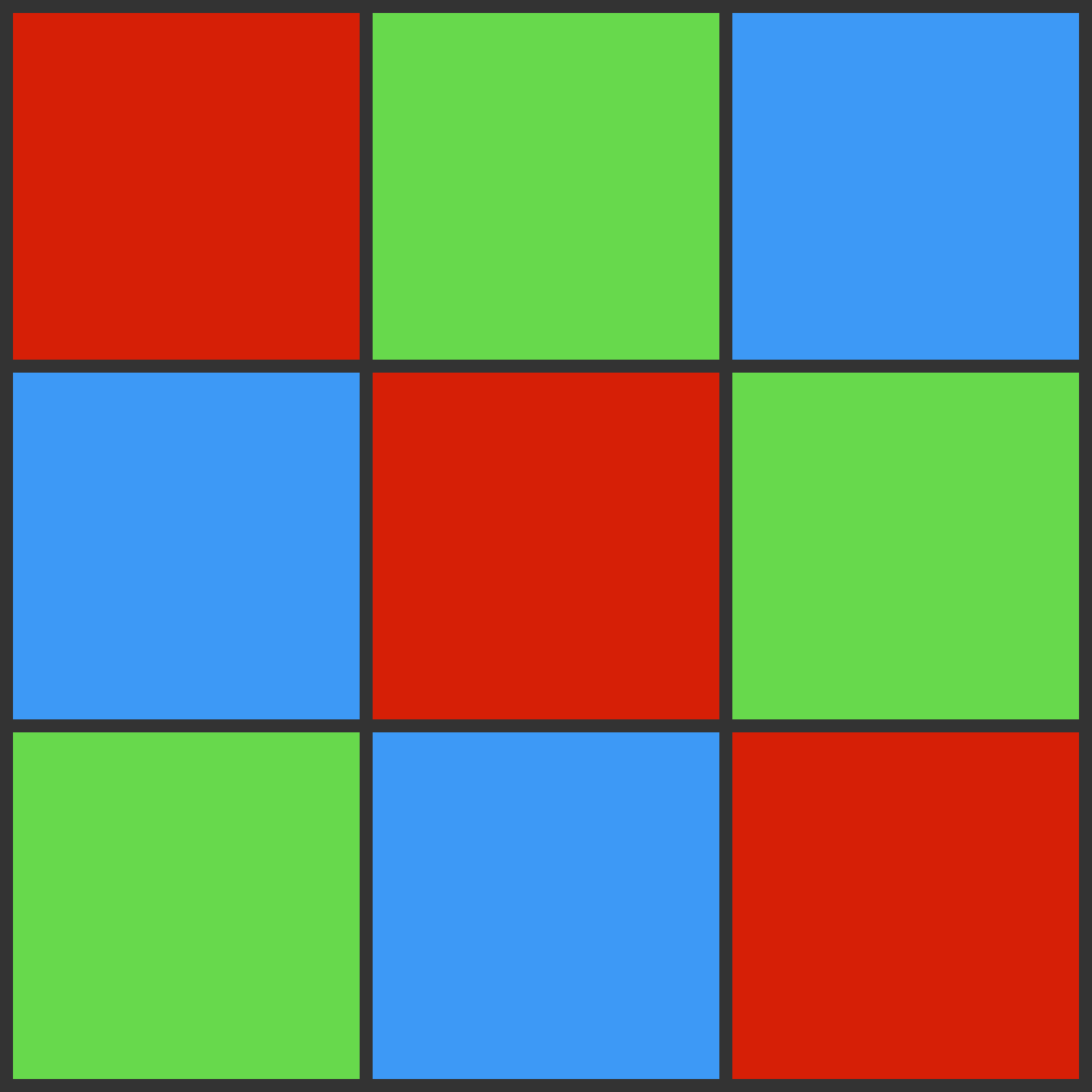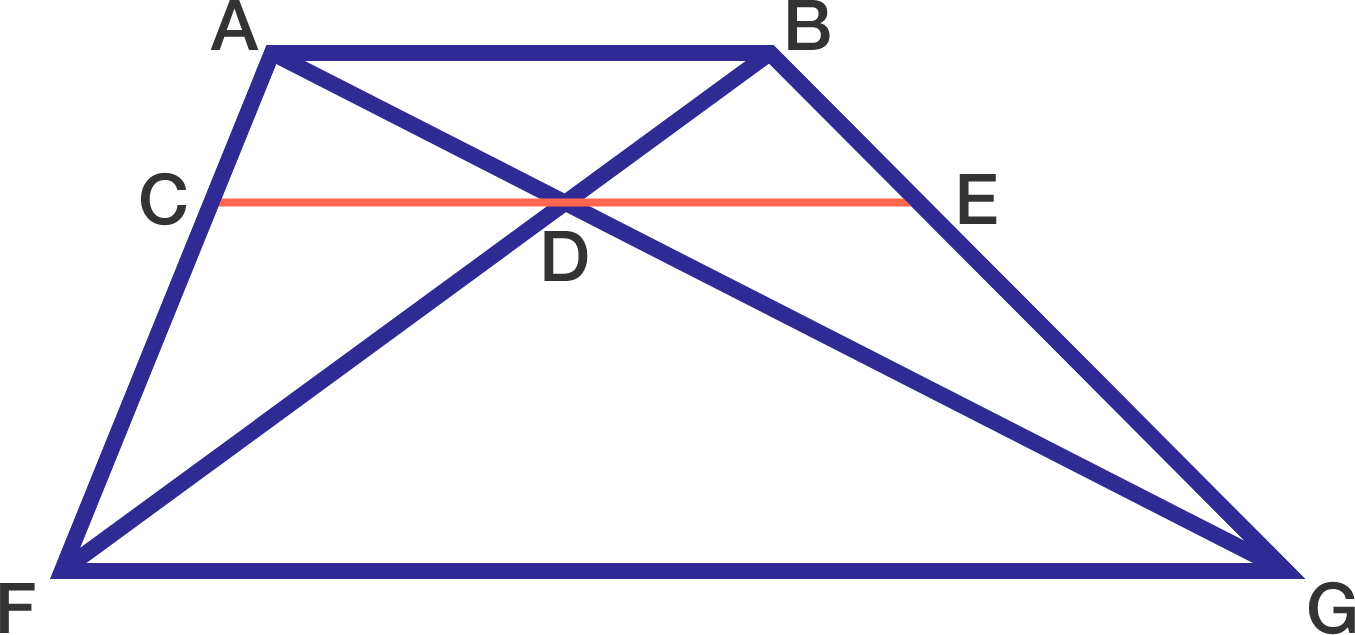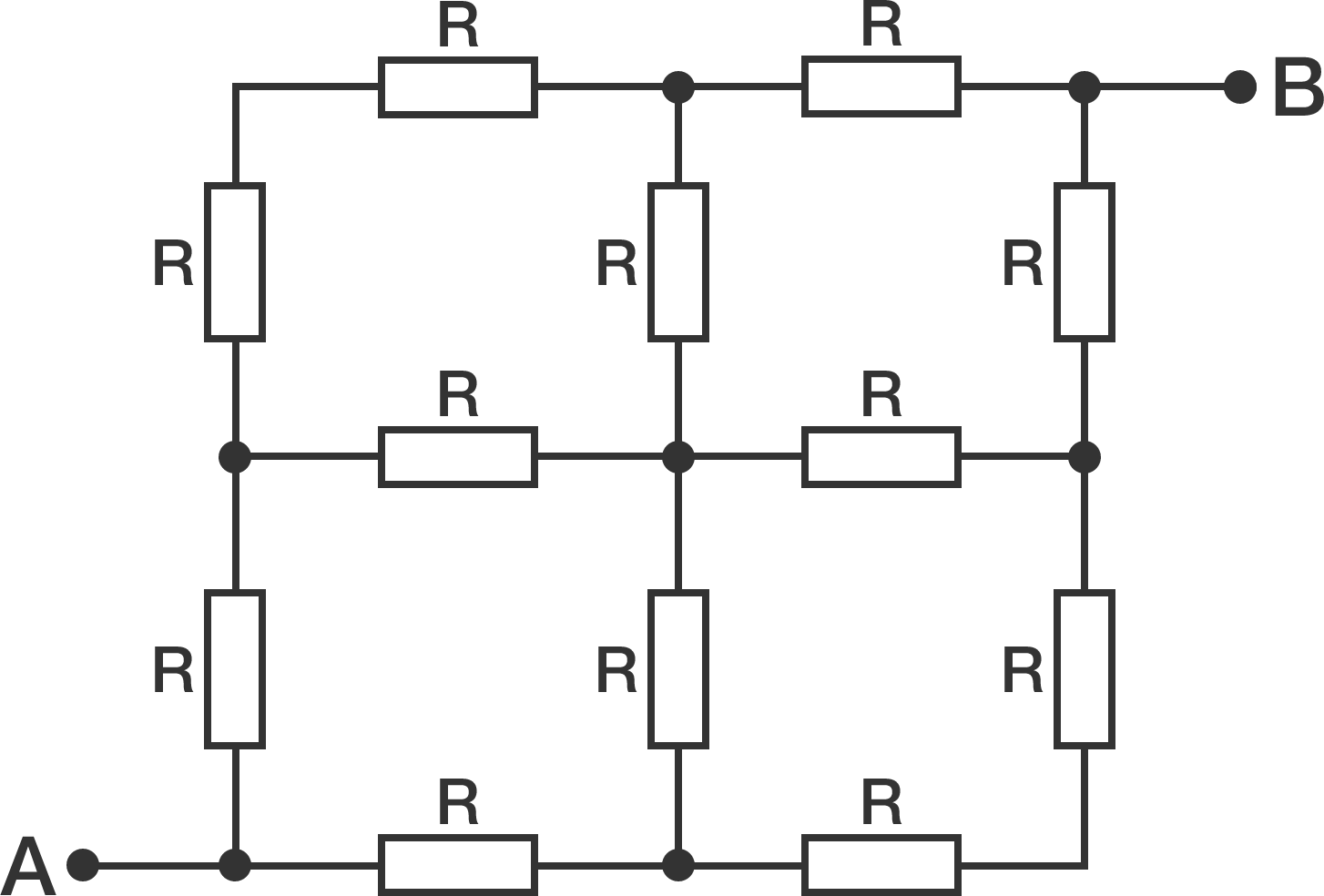# Problems of the Week

Contribute a problem

# 2017-05-22 Intermediate

If $y^2 -4y = x^2 - 4x,$ then does it necessarily follow that $y= x?$How many ways are there to paint a $3\times3$ grid with three colors such that each row and column has all three distinct colors?

Note: If you rotate the above illustration by $90^\circ, 180^\circ$ or $270^\circ$, they each count as different ways to paint the $3\times3$.

In the diagram below, $ABGF$ is a trapezoid and $D$ is the intersection point of its diagonals.

If $CE$ is drawn such that it passes through $D$ and is parallel to $AB,$ is it true that $CD = DE?$In a small, remote country, there are only two types of bills--the $X bill and the$Y bill.

With combinations of these bills, almost all positive integer prices can be paid exactly. Only fifteen positive integer prices can't be paid exactly, one of which is \$18.

What is $X+Y?$All the resistors in the above circuit have equal resistance $R = \SI[per-mode=symbol]{10}{\ohm}$. Calculate the equivalent resistance $R_e$ between points $A$ and $B$ in ohms. Submit your answer to the nearest integer.

×